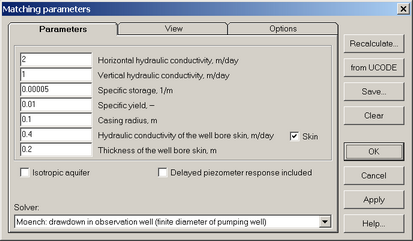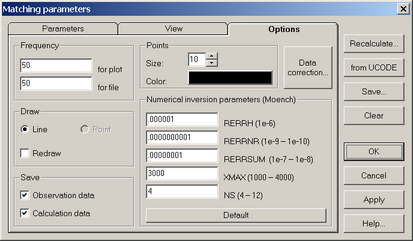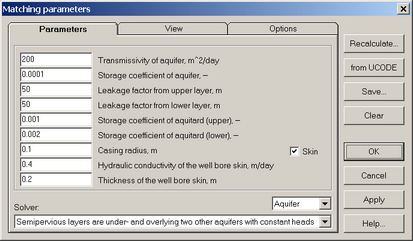﻿ ANSDIMAT+ > Aquifer tests analysis > Matching parameters (solving direct problem) > Value assignment

# Value assignment

In the "Matching parameters" dialog box, assumed flow equation parameters are specified. These parameters are applied for solving the direct problem. The calculated results (curves) are visually compared to observation data. For each conceptual model, a specific set of parameters is determined. For some models where different equations can be applied, it is possible to select a specific solution. This provides the opportunity to analyze and compare calculated drawdown values obtained from different solutions.

The dialog box opens from the main menu "Analysis > Direct solution", function key F7 or by right click in the lower left corner of the"Matching parameters" dialog boxes."Matching parameters" dialog boxes: examples .

Explanatory table

 "Parameters" tab Specifies aquifer parameters for matching and choosing the solutionText boxes for flow equation coefficients input. The number of boxes and their names depend on the chosen conceptual model "Isotropic aquifer" option Specifies vertical anisotropy (for models with partially penetrating wells) "Delayed piezometer reponse included" option Includes storage capacity and shape factor of piezometer in drawdown calculations (for some of the Moench’s solutions) "Skin" option Uses skin-factor for matching parameters List Selects piezometer location for matching parameters "Solver" list box Selects a solution for matching parameters. Occurs if the conceptual model can be analyzed using several solutions "View" tab Defines the theoretical curve appearance "Options" tab Options for data displaying and saving "Frequency" frame Specifies the precision of the plot (the number/frequency of calculation points within the entire calculation interval) and the frequency of calculation points saved in a file by clicking the "Save" button "Draw" frame Options for displaying calculation data "Lines" option In the current version of the program the displayed curve has only the straight-line view "Redraw" option Switches on/off the updating option for calculated curves (when pressing "OK" or "Apply" buttons) "Save" frame Choose the values (either observed or/and calculated), displayed on the current plot, for saving (when pushing "Save" button) "Points" frame Sets-up the appearance of calculation points on distance-drawdown plots "Size" text box Dot diameter "Color" button Dot color "Data correction" button Opens dialog box "Data correction" "Numerical inversion parameters(Moench)" frame Parameters for Moench’s solutions. Applied in case of Moench’s solution instability (see WTAQ2 and WTAQ3 program description). The input values are saved automatically. Press "Default" button to return to initial values "RERRH" text box Relative error criterion for Hantush convergence (for WTAQ2 only); in the program the parameter is only used with Moench’s solutions for linear source in confined aquifer "RERRNR" text box Relative error for Newton-Raphson iteration and summation (WTAQ2: 1e-10; WTAQ3: 1e-9 to 1e-10) "RERRSUM" text box Relative error for summation (WTAQ2: 1e-15; WTAQ3: 1e-7 to 1e-8) "XMAX" text box Maximum number of terms in summation (WTAQ2: 1000 – 4000; WTAQ3: 3000) "NS" text box Number of terms used in the Stehfest algorithm. This must be an even number. If the computer holds 16 significant figures in double precision, NS should be between 8 and 12. Note: if results are numerically unstable, as may occur if beta is very small, reduced to 6 (or even 4). In the latter case, precision will be reduced, however, and results should be checked for accuracy "Default" button Specifies parameter values for WTAQ3 program "Recalculate" button Recalculates aquifer parameters (see "Parameter calculation" dialog box description); specifies piezometer and observation well shape factors for Moench’s solutions "from UCODE" button Loads parameters from the previously solved inverse problem "Save" button Saves observed and/or calculated parameter values in dat file "Clear" button Updates the plot. Previously plotted calculated curves are deleted